## Loren on the Art of MATLABTurn ideas into MATLAB

Note

Loren on the Art of MATLAB has been archived and will not be updated.

# Intersecting Lines (Part 2)

Recently, Lucio and I wrote a post on detecting line segment intersections. We confessed in the post that we had not been exhaustive in our treatment. We also received many interesting comments. This post introduces a 3rd method, one that several readers had mentioned; the post includes several variations to deal with various edge cases as cleanly and numerically soundly as possible, particularly ones related to having a vertical line segment, i.e., a segment with infinite slope.

### Third Algorithm to Account for Vertical Segments

The previous two algorithms fail when there is a vertical line segment. Some research on the web indicates that one of the preferred solutions for this problem is to parameterize the line segments as two vectors. Several of our blog readers have already commented about this approach.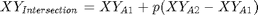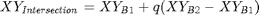where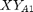and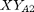(2x1) indicate the coordinates of the endpoints of line A,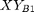and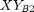(2x1) indicate the coordinates of the endpoints of line B,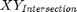indicates the coordinates of the intersecting point (if one exists) andandare unknown scalars.

This leads to a system of equations; testing for an intersection consists on solving forandand checking that both are in [0,1]. Note that we use linsolve rather than left matrix divide (or inv) as linsolve has better numerical properties when the system of equations is ill-conditioned.

A = @(lineA,lineB) [lineA(1,:) - lineA(2,:); lineB(2,:) - lineB(1,:)]';
pq = @(lineA,lineB) linsolve(A(lineA,lineB),(lineB(2,:) - lineA(2,:))');
isIntersect = @(lineA,lineB) all(all(pq(lineA,lineB)*[1 -1]+[0 1;0 1]>=0));

where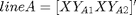and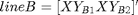(x-coordinates are the first column and y-coordinates are the second column, as in our previous post).

In order to simplify our writing, we put all plot commands inside a utility function:

dbtype plotlines
1     function plotlines(line1, line2)
2     % Plots two line segments and annotates the endpoints
3       figure
4       hold all
5       h(1) = plot(line1(:,1),line1(:,2),'b^-');
6       h(2) = plot(line2(:,1),line2(:,2),'rs-');
7       set(h,'linewidth',2)
8       g(1) = text(line1(1,1),line1(1,2),...
9           sprintf('  (%1.20g,%1.20g)  ',line1(1,1),line1(1,2)),...
10          'verticalalignment','top','color','b');
11      g(2) = text(line1(2,1),line1(2,2),...
12          sprintf('  (%1.20g,%1.20g)  ',line1(2,1),line1(2,2)),...
13          'verticalalignment','top','color','b');
14      g(3) = text(line2(1,1),line2(1,2),...
15          sprintf('  (%1.20g,%1.20g)  ',line2(1,1),line2(1,2)),...
16          'verticalalignment','bottom','color','r');
17      g(4) = text(line2(2,1),line2(2,2),...
18          sprintf('  (%1.20g,%1.20g)  ',line2(2,1),line2(2,2)),...
19          'verticalalignment','bottom','color','r');
20      ext = cell2mat(get(g,'Extent'));
21      axis([min(ext(:,1)),max(ext(:,1)+ext(:,3)),...
22          min(ext(:,2)),max(ext(:,2)+ext(:,4))])
23      hold off
24    end


Now we test the new algorithm with two intersecting segments with one of them having infinite slope:

lineA = [.5 1; ...
.5 0];
lineB = [0.6 0.5; ...
0 0.5];
plotlines(lineA,lineB)
plotlines(lineB,lineA)

isIntersect(lineA,lineB)
isIntersect(lineB,lineA)
ans =
1
ans =
1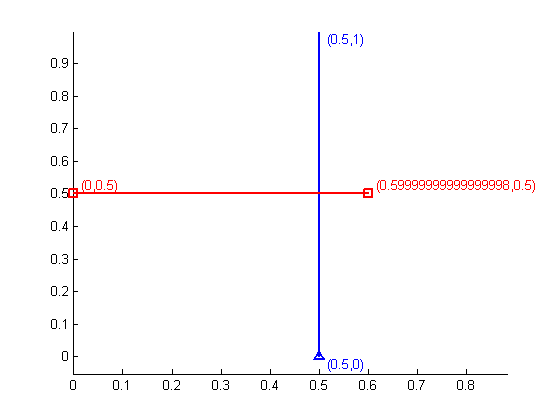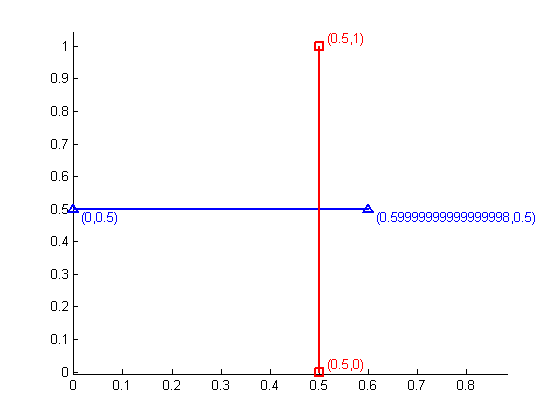Test with two non-intersecting segments:

lineA = [.5 1; ...
.5 0];
lineB = [0.4 0.5; ...
0 0.5];
plotlines(lineA,lineB)
plotlines(lineB,lineA)
isIntersect(lineA,lineB)
isIntersect(lineB,lineA)
ans =
0
ans =
0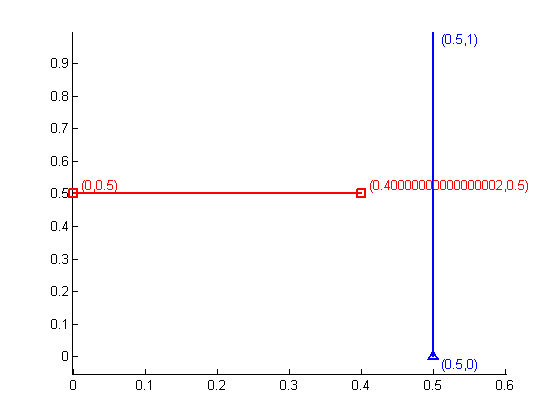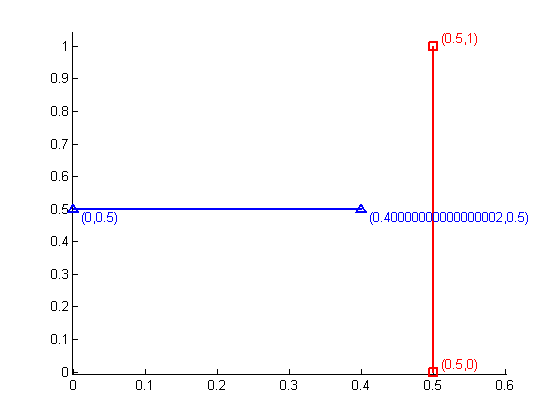### Add Tolerance for Rounding Errors

In many applications, the computer numerical precision is not sufficient to represent an exact quantity; therefore special care needs to be taken when testing if an intersection point exists. For example observe the following inconsistency with our current algorithm:

lineA = [0 0; ...
1 3];
lineB = [1/3 1; ...
0 1];
lineC = [1/3 1; ...
1 1];
plotlines(lineA,lineB)
isIntersect(lineA,lineB)
plotlines(lineA,lineC)
isIntersect(lineA,lineC)
ans =
0
ans =
1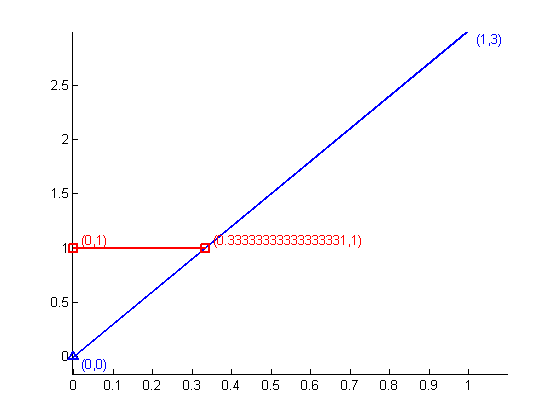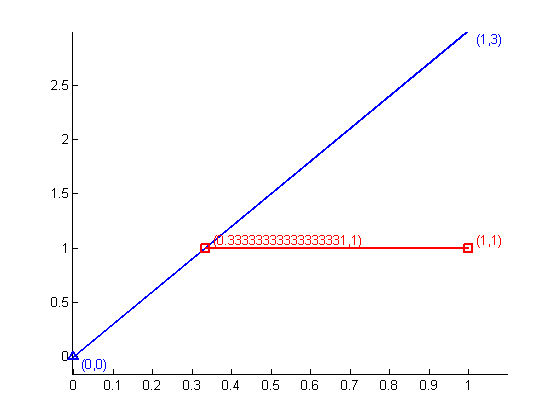One endpoint for both lineB and lineC lies on the segment lineA. This should result in an intersection in both cases. We can fix this problem by introducing a tolerance in our final testing. A conservative tolerance to use is sqrt(eps), however one may prefer to do something more robust that depends on the values involved in the computations. This has already been discussed in a previous blog post.

isIntersect = @(lineA,lineB) all(all(pq(lineA,lineB)*[1 -1]+[0 1;0 1]>=-sqrt(eps)));
isIntersect(lineA,lineB)
isIntersect(lineA,lineC)
ans =
1
ans =
1


### Detecting Parallel Lines

One of the nice properties of the formulation we are using is that parallel lines (segments with the same slope) can be easily detected by testing if A is singular. This can be calculated by checking that det(A)==0. For example:

lineA = [0 0; ...
1 1];
lineB = [1 2; ...
2 3];
plotlines(lineA,lineB)
det(A(lineA,lineB))
ans =
0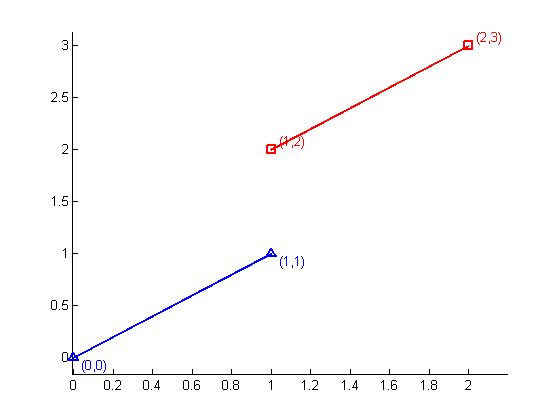The astute reader will promptly notice that this straightforward calculation is also vulnerable to numerical precision problems, for instance:

lineC = [0 1; ...
1 1/3];
lineD = [1 2/3; ...
2 0];
plotlines(lineC,lineD)
det(A(lineC,lineD))
ans =
-1.1102e-016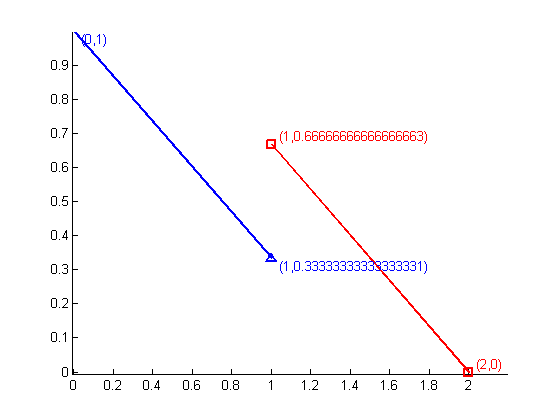The proper way to test for singularity is to use the function rank, as it incorporates proper tolerances to overcome rounding errors.

isParallel = @(lineA,lineB) rank(A(lineA,lineB))<2;
isParallel(lineA,lineB)
isParallel(lineC,lineD)
ans =
1
ans =
1


We now incorporate the test for parallel lines. For clarity, we now created a function in a file rather than an anonymous function.

dbtype isIntersect2
1     function tf = isIntersect2(lineA, lineB)
2     A = [lineA(1,:) - lineA(2,:); lineB(2,:) - lineB(1,:)]';
3     if rank(A) < 2
4         disp('Parallel')
5         tf = false;
6     else
7         pq = linsolve(A,(lineB(2,:) - lineA(2,:))');
8         tf = all(pq>=-sqrt(eps)) & all(pq<=1+sqrt(eps));
9     end

isIntersect2(lineA,lineB)
isIntersect2(lineC,lineD)
Parallel
ans =
0
Parallel
ans =
0


### Testing for Collinearity

Collinearity is a special case of parallel lines; when this occurs, it the line segments might overlap. In a previous blog post, we have already talked about testing for collinearity; we'll incorporate our previous findings here. Notice that we also use rank for numerical stability. Also observe that since collinearity implies parallel segments, we only need to test any three end-points of the two line segments for collinearity. In our implementation the second endpoint of lineB is not used for testing collinearity.

lineA = [0 1; ...
1 1/3];
lineB = [2.5 -2/3; ...
1.5 0];

plotlines(lineA,lineB)
dbtype isIntersect3
1     function tf = isIntersect3(lineA, lineB)
2     A = [lineA(1,:) - lineA(2,:); lineB(2,:) - lineB(1,:)]';
3     if rank(A) < 2
4         disp('Parallel')
5         B = [lineA(1,:) - lineA(2,:); lineA(1,:) - lineB(1,:)]';
6         if rank(B) < 2
7             disp('Collinear')
8             tf = NaN;
9         else
10            tf = false;
11        end
12    else
13        pq = linsolve(A,(lineB(2,:) - lineA(2,:))');
14        tf = all(pq>=-sqrt(eps)) & all(pq<=1+sqrt(eps));
15    end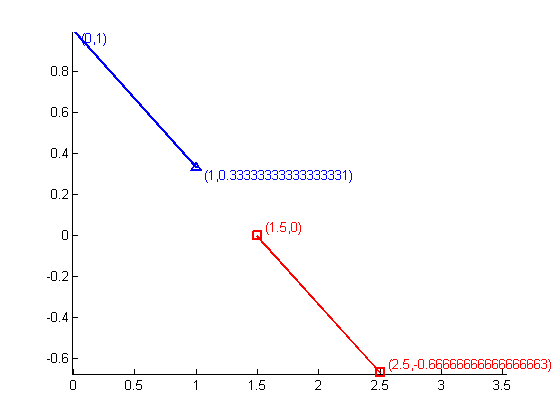isIntersect3(lineA,lineB)
Parallel
Collinear
ans =
NaN


Once we confirm that two segments are collinear, then the segments can be projected either to the x- or y-axis. We now need to determine if the endpoints of the segments are interleaved or not, to figure out if they overlap and hence intersect. Once again, a tolerance must be incorporated into the testing.

To check if the x-coordinates of the points are interleaved, we implement the following tests; both inequalities must be false in order to discard an intersection (or segment overlapping):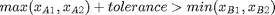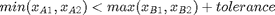dbtype isIntersect4
1     function tf = isIntersect4(lineA, lineB)
2     A = [lineA(1,:) - lineA(2,:); lineB(2,:) - lineB(1,:)]';
3     if rank(A) < 2
4         disp('Parallel')
5         B = [lineA(1,:) - lineA(2,:); lineA(1,:) - lineB(1,:)]';
6         if rank(B) < 2
7             disp('Collinear')
8             if all( (sort(lineA(:,1),'descend')-sort(lineB(:,1))) ...
9                     .*[-1;1] <= sqrt(eps) )
10                tf = true;
11            else
12                tf = false;
13            end
14        else
15            tf = false;
16        end
17    else
18        pq = linsolve(A,(lineB(2,:) - lineA(2,:))');
19        tf = all(pq>=-sqrt(eps)) & all(pq<=1+sqrt(eps));
20    end


isIntersect4(lineA,lineB)
Parallel
Collinear
ans =
0

lineA = [0 1; ...
1-2*eps 1/3-eps];
lineB = [1+2*eps 1/3; ...
1.5 eps];
plotlines(lineA,lineB)
isIntersect4(lineA,lineB)
Parallel
Collinear
ans =
1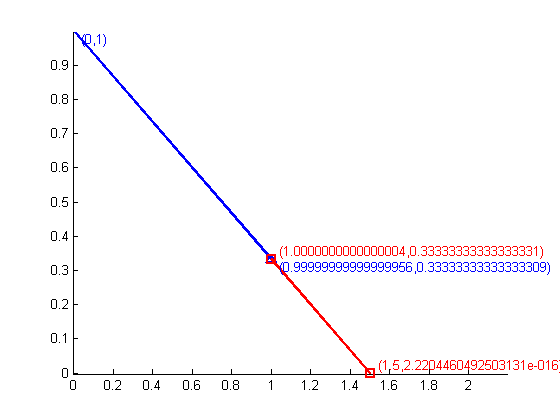### Degenerate Lines

Observe that the tests for singularity and collinearity take care properly of the degenerate lines:

lineA = [0 0;2 2];
lineB = [1 1;1 1];
lineC = [1 2;1 2];
lineD = [2 2;2 2];
plotlines(lineA,lineB)
isIntersect4(lineA,lineB)
plotlines(lineA,lineC)
isIntersect4(lineA,lineC)
plotlines(lineB,lineD)
isIntersect4(lineB,lineD)
Parallel
Collinear
ans =
1
Parallel
ans =
0
Parallel
Collinear
ans =
0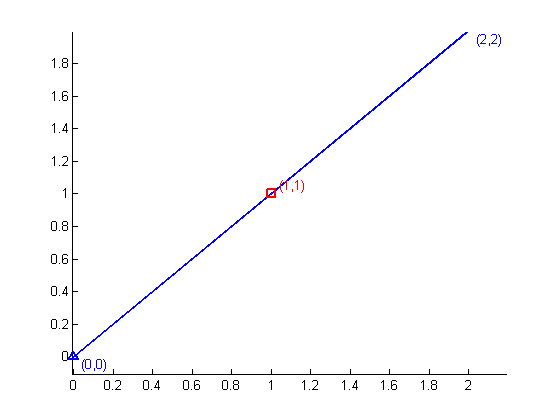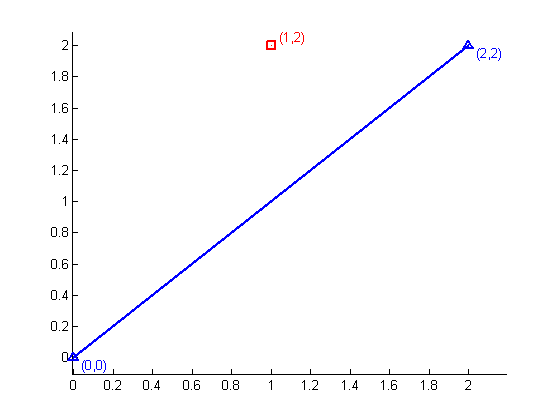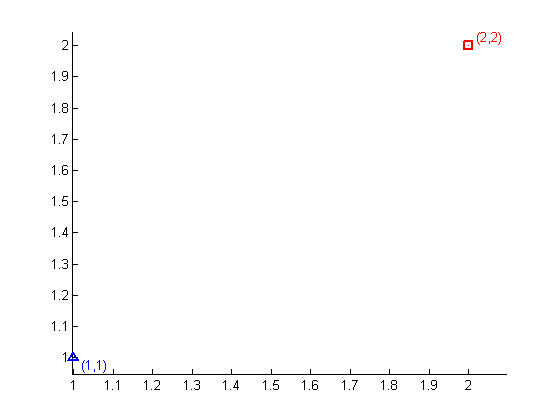We are almost done, however there is one edge case that will still break our algorithm. Can you identify it (HINT: it is not associated with our conservative tolerances)? Let us know what you find here.

Published with MATLAB® 7.13

### 댓글

댓글을 남기려면 링크 를 클릭하여 MathWorks 계정에 로그인하거나 계정을 새로 만드십시오.